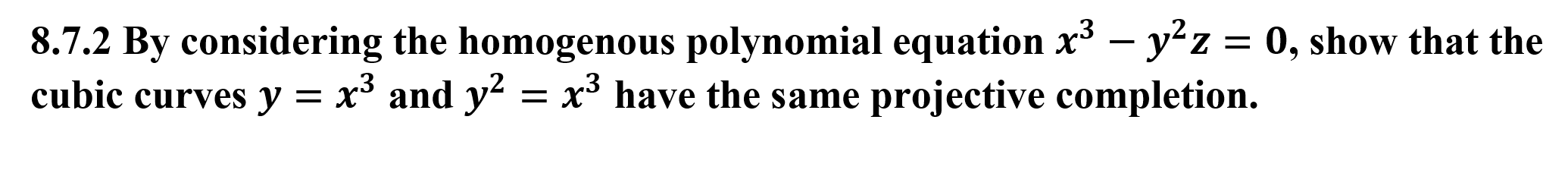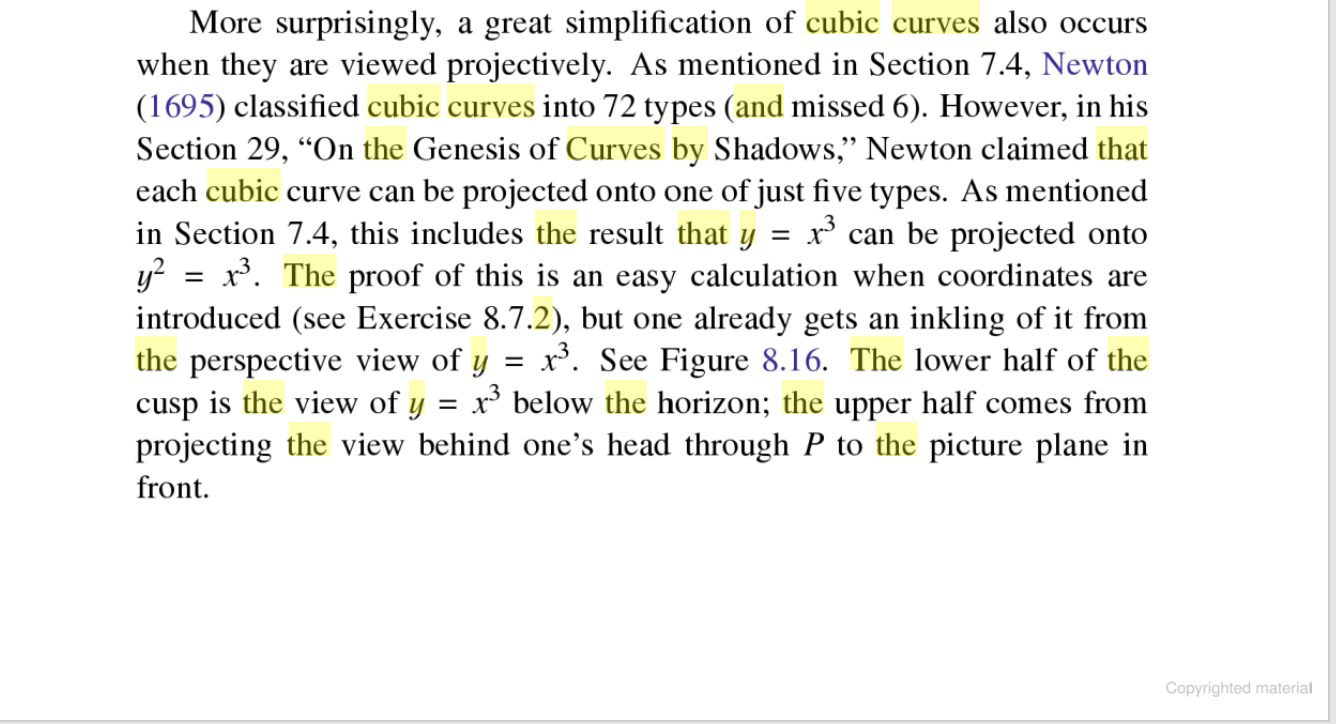# 8.7.2 By considering the homogenous polynomial equation x3 - yz = 0, show that thecubic curves y = x5 and y2 = x3 have the same projective completion. More surprisingly, a great simplification of cubic curves also occurswhen they are viewed projectively. As mentioned in Section 7.4, Newton(1695) classified cubic curves into 72 types (and missed 6). However, in hisSection 29, "On the Genesis of Curves by Shadows," Newton claimed thateach cubic curve can be projected onto one of just five types. As mentionedin Section 7.4, this includes the result that yy x. The proof of this is an easy calculation when coordinates areintroduced (see Exercise 8.7.2), but one already gets an inkling of it fromthe perspective view of ycusp is the view of yprojecting the view behind one's head through P to the picture plane infrontx can be projected ontox. See Figure 8.16. The lower half of thex3 below the horizon; the upper half comes fromCopyrighted material

Questionhelp_outlineImage Transcriptionclose8.7.2 By considering the homogenous polynomial equation x3 - yz = 0, show that the cubic curves y = x5 and y2 = x3 have the same projective completion. fullscreenhelp_outlineImage TranscriptioncloseMore surprisingly, a great simplification of cubic curves also occurs when they are viewed projectively. As mentioned in Section 7.4, Newton (1695) classified cubic curves into 72 types (and missed 6). However, in his Section 29, "On the Genesis of Curves by Shadows," Newton claimed that each cubic curve can be projected onto one of just five types. As mentioned in Section 7.4, this includes the result that y y x. The proof of this is an easy calculation when coordinates are introduced (see Exercise 8.7.2), but one already gets an inkling of it from the perspective view of y cusp is the view of y projecting the view behind one's head through P to the picture plane in front x can be projected onto x. See Figure 8.16. The lower half of the x3 below the horizon; the upper half comes from Copyrighted material fullscreen
check_circleExpert Solution
Step 1

To show that the  two given affine cubic curves have the same projective completion

Step 2

The equations y=x^3 and y^2=x^3 represent two different curves in the affine plane (cartesian coordina...

### Want to see the full answer?

See Solution

#### Want to see this answer and more?

Solutions are written by subject experts who are available 24/7. Questions are typically answered within 1 hour*

See Solution
*Response times may vary by subject and question
Tagged in

### Math